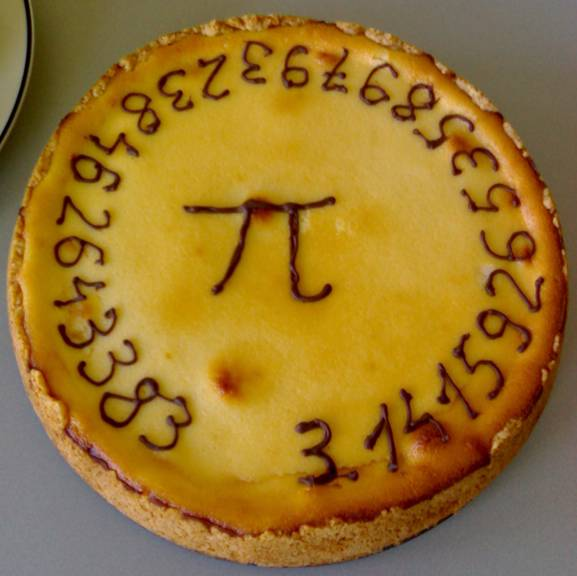### Archive

Posts Tagged ‘casino’

## Happy Pi Day Everyone!Yes March 14 (3/14) is indeed pi day; a day to celebrate everyone’s favourite irrational number.

As a special treat for you, I’m going to show you a way to calculate pi to a high accuracy for yourself (knowledge of a computer programming language helps).

So the number pi is closely related to the geometry of the circle. The area of a circle is represented by the following formula:

Where r is the radius of the circle. So we can use this relationship to calculate pi, but we can do it without measuring anything! Instead, we are going to use something called a “Monte Carlo” simulation

Monte Carlo simulations are very useful in physics. They use random numbers to simulate a real-world system. Since they are based on random numbers, the technique is named after the famous Monte Carlo casino.

Ok, so imagine you have a square that is 1 meter by 1 meter. Now imagine you place one-quarter of a circle with a radius of 1 meter over top of this square, like so:Since the radius of the circle is 1 meter, and we are looking at exactly one-fourth of the complete circle, the area of this “slice” of circle is:The area of the square isAnd since it is 1 meter in length and 1 meter in width, the area is simply 1 meter squared:Since the area of the square is simply 1, the ratio of the area of the square to the area of the slice is:So we can see that pi is directly related to the ratio of the area of the slice to the area of the square.

Don’t worry, I’m goin’ somewhere with this…

Now comes the Monte Carlo part of the problem. We are going to generate 2 random numbers between 0 and 1. The first random number will be an x-coordinate, and the second will be a y-coordinate. For illustrative purposes, lets say the numbers turned out to be 0.5 and 0.5. So lets put a red circle at (0.5, 0.5):We can see that this point lies inside the circle. In fact, any x and y values which satisfy this equation:will lie inside the circle.So what if we generated a huge number of points and counted the number that landed inside the circle? If we did this, then the ratio of the number of points which landed inside to circle to the total number of points would be:Which you see is exactly the same as the equation above which related the area of the slice to the area of the square, except for one little difference. The “squiggly line” equal sign in the above equation means “approximately equal to”. It indicates that this method is an approximation because we are only using a finite number of points. If we generated an infinite number of points, that “approximately equal” sign will change to a regular equal sign.

So now all we have to do is run this calculation for a large number of points (N) and we will find that:So how accurate is this method? Well again it will depend on the number of points. For example, if I run this method using 10 points I get a value for π of about 3.6.

If I use N = 100 points I get π ~ 3.16

And if I use N = 1 000 000, I get π = 3.140916

which is getting pretty close to the actual value of about 3.1415926539…

There you go. I’m sure your life will be much richer and meaningful now that you have this information. Now if you’ll excuse me, I’m going to eat some pie.

Categories: Physics